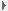[an error occurred while processing this directive] 高校地质学报 2002, 8(3) 241-247 DOI:     ISSN: 1006-7493 CN: 32-1440/P

 扩展功能 本文信息Supporting infoPDF(1278KB)[HTML全文]参考文献[PDF]参考文献 服务与反馈把本文推荐给朋友加入我的书架加入引用管理器引用本文Email Alert文章反馈浏览反馈信息 本文关键词相关文章 本文作者相关文章徐世浙戴世坤兰翔 PubMedArticle byArticle byArticle by

1. 浙江大学地球科学系，杭州 310027; 2. 石油大学地球科学系，北京 102200

A New Fast Method for Continuation of Potential Field on an Undulate Terrain
XU Shi-zhe1, DAI Shi-kun2, LAN Xiang1
1. Department of Geosciences, Zhejiang University, Hangzhou 310027, China; 2. Department of Geosciences, Petroleum University, Beijing 102200, China
Abstract: Vertically projecting the observation point at a undulate terrain onto a horizontal plane and copying the potential at the terrain to the corresponding point at the horizontal plane,we get a fictitious potential field on a horizontal plane.An FFT is then can applied to calculate the outward normal derivativ a u／ n of the potential u on the horizontal plane.The calculated derivativeis u／ n is used approximately as the outward normal derivative of u／ n of the potential on the terrain.Based on the potential u and the normal derivativeon the terrain,Green’s formula is used to calculate the potential u at an arbitrary point above the terain.When the potential u at a level above the terrain is obtained,an FFT is used again to calculate the potential u at other level above the sources of the potentia1.The other method of continuation of potential field,e.g.,the equivalent source method, requires solving a large set of linear algebraic equations that take a hefty amount of computer memory and expends considerably the computation time.This method does not require solving algebraic equations and is of a high computation speed.The model tests demonstrated that the accuracy of this method is satisfactory.Using this method to continue the practical aeromagnetic anomaly shows a good result.
Keywords:

DOI: